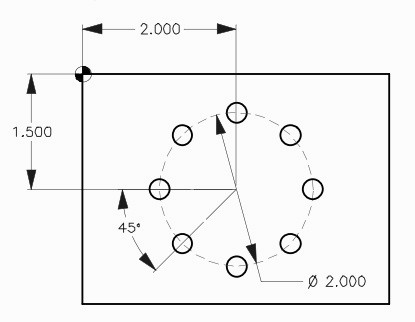# CNC Milling G70 Bolt Hole Circle Program Example

G70 Bolt Hole Circle command makes it easy to drill/tap/bore in a circle at even distances.
There is no need to know or calculate each hole position.
You just are required to know is the Radius of the circle, Starting angle of the first hole and Total number of holes.

## G70 Bolt Hole Circle Format

`G70 I J L`

I = Radius of the bolt hole circle (+CCW / – CW).
J = Starting angle of first hole ( 0 to 360° CCW from horizontal at the 3 o’clock position).
L = Number of evenly spaced holes around bolt hole circle.

## CNC Milling G70 Bolt Hole Circle Program Example

Following cnc program example uses G81 Drilling Cycle in combination with G70 Bolt Hole Circle G-code to drill 8 holes evenly spaced in a circle of 1 (inch) radius.G70 Bolt Hole Circle Program Example

```O0009
N1 T1 M06
N2 G90 G54 G00 X2. Y-1.5  (Center position of bolt hole circle)
N3 S1451 M03
N4 G43 H01 Z1. M08
N5 G81 G99 Z-0.45 R0.1 F8. L0
N6 G70 I1. J0. L8
N7 G80 G00 Z1. M09
N8 G53 G49 Z0. M05
N9 M30```